International
Tables for
Crystallography
Volume F
Crystallography of biological macromolecules
Edited by M. G. Rossmann and E. Arnold

International Tables for Crystallography (2006). Vol. F, ch. 19.5, pp. 445-446   | 1 | 2 |

Section 19.5.3. Diffraction by helical molecules

R. Chandrasekarana* and G. Stubbsb

aWhistler Center for Carbohydrate Research, Purdue University, West Lafayette, IN 47907, USA, and  bDepartment of Molecular Biology, Vanderbilt University, Nashville, TN 37235, USA
Correspondence e-mail:  chandra@purdue.edu

19.5.3. Diffraction by helical molecules

| top | pdf |

19.5.3.1. Fibre diffraction patterns

| top | pdf |

As noted above, the diffraction pattern from a fibre is confined to layer lines because of the repeating nature of the polymer helix. The layer lines in reciprocal space are perpendicular to the fibre axis in real space. The layer line passing through the origin in reciprocal space is called the equator or zero layer line. The line in a diffraction pattern normal to the equator and passing through the origin is called the meridian. If the fibre axis is perpendicular to the incident X-ray beam, the recorded diffraction pattern is symmetric about both the equator and the meridian. If the fibre is not normal to the incident beam, the pattern is symmetric only about the meridian.

19.5.3.2. Helical symmetry

| top | pdf |

It is convenient to use cylindrical coordinates to describe helical molecules. In real space we use coordinates (r, ϕ, z); in reciprocal space (R, ψ, Z). By convention, the z axis is the helix axis and the line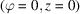corresponds to the x axis in Cartesian coordinates. The repeat distance along the z axis is c. Within this distance, there are u repeating units in t turns of the helix. If the coordinates of a point in the first repeating unit are (r, ϕ, z), then applying the helical symmetry gives the coordinates of the corresponding point in the (k + 1)th repeating unit as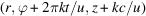.

19.5.3.3. Structure factors

| top | pdf |

Cochran et al. (1952)showed that the structure factor on layer line l of a helix made up of repeating subunits is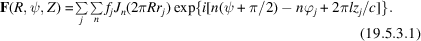Diffraction occurs only for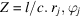and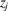are the real-space coordinates of atom j in the repeating unit of the helix;is the atomic scattering factor of that atom.is the Bessel function of the first kind of order n. The summation over n includes only those values of n that satisfy the selection rule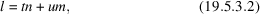where m is any integer. In practice, the summation may be limited to values ofless than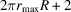, whereis the radius of the outermost atom in the polymer, because the value of a Bessel function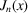is negligible for n greater than about x + 2. For low-order Bessel functions or applications requiring greater accuracy, slight variations of this limitation are used.

The structure factor F is a complex number with an amplitude and phase, and is fully equivalent to that derived using the trigonometric functions in crystallography. The expression for intensity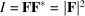holds good.

Equation (19.5.3.1) can be rewritten (Klug et al., 1958) as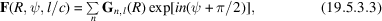where the Fourier–Bessel structure factor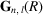is independent of ψ and is given by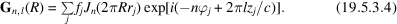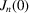is 1 whenand 0 otherwise. For this reason, the structure factors on the meridian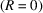are nonzero only on layer lines for which l is an integral multiple of u. Hence, a visual inspection of the diffraction pattern often helps to determine u.

19.5.3.4. Fourier–Bessel syntheses

| top | pdf |

Electron densities may be calculated for crystalline fibres, as they are in crystallography, using Fourier syntheses with coefficients determined for the crystalline reflections.

For noncrystalline fibres, it is more convenient to use Fourier–Bessel syntheses: the electron density ρ at pointis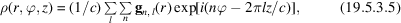where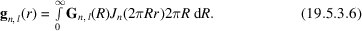19.5.3.5. Diffracted intensities: noncrystalline fibres

| top | pdf |

The intensity in the diffraction pattern of a noncrystalline fibre is the cylindrical average of the square of the Fourier transform (Franklin & Klug, 1955):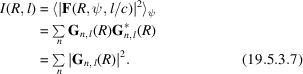The intensity varies continuously as a function of R along each layer line (Fig. 19.5.2.1a).

19.5.3.6. Diffracted intensities: polycrystalline fibres

| top | pdf |

The intensity in the diffraction pattern of a polycrystalline fibre consists of Bragg reflections on layer lines (Fig. 19.5.2.1b). On each layer line, owing to the lattice sampling that arises from the lateral organization of the polymers, intensities are observed at discrete R values defined by the reciprocal-lattice points. In the case of monoclinic (with c as the unique axis), orthorhombic and hexagonal systems, the reflection positions are determined by equations (19.5.3.8), (19.5.3.9) and (19.5.3.10), respectively.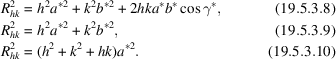Consequently, on each layer line, superposition occurs between reciprocal-lattice points (hkl) and () for monoclinic; (hkl), (), () and () for orthorhombic; and (hkl), (), (khl), (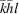), (kil), (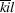), (ikl), (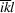), (ihl), (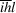), (hil) and (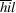), where, for hexagonal systems. Depending upon the unit-cell dimensions, other reflections having the same R value may also be superposed to give a single intensity, and those having R values close to each other may be difficult to resolve. All superposed reflections must be considered individually when calculating such composite intensities.

References

Cochran, W., Crick, F. H. & Vand, V. (1952). The structure of synthetic polypeptides. I. The transform of atoms in a helix. Acta Cryst. 5, 581–586.Google Scholar
Franklin, R. E. & Klug, A. (1955). The splitting of layer lines in X-ray fibre diagrams of helical structures: application to tobacco mosaic virus. Acta Cryst. 8, 777–780.Google Scholar
Klug, A., Crick, F. H. C. & Wyckoff, H. W. (1958). Diffraction from helical structures. Acta Cryst. 11, 199–212.Google Scholar